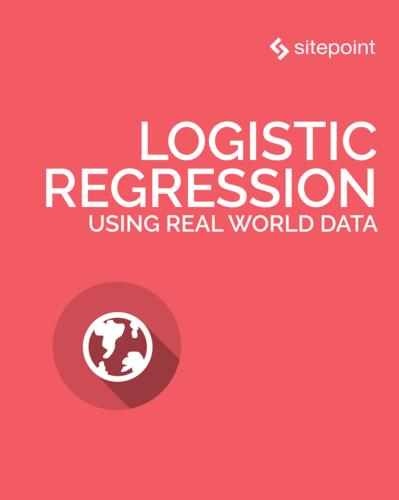Preview

# Logistic Regression Using Real-world Data

#### What’s included in SitePoint Premium?

• 250+ books
• 120+ courses
• Cancel anytime
• 30 Days Money Back Guarantee!

### Description:

Logistic regression is an extension of the linear regression model designed to predict the probability of occurrence for a binary outcome. As such, it lends itself rather well to binary classification tasks and can potentially be extended to multi-class problems using more advanced techniques not discussed in this tutorial.

Examples of problems that logistic regression can solve include how likely someone is to develop lung cancer given their age, weight, and cigarette intake. Or a conversion model that predicts how likely it is that a lead will convert into a paying customer based on their income, demographics, and time since our most recent contact.

Alongside just making predictions, logistic regression is also an excellent choice for descriptive analytics and quantifying the relationship between a variable and the response. For example, what effect does smoking one more packets of cigarettes have on the probability of getting lung cancer? Because of its simple mathematical form and ease of interpretation, logistic regression is a great tool for tackling such questions where explainability is a major concern (for example, in econometrics research).

This tutorial will begin with a brief introduction to the mathematical form of logistic regression and then use the technique to detect fraud in a synthetic financial transactions dataset.

available in: